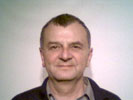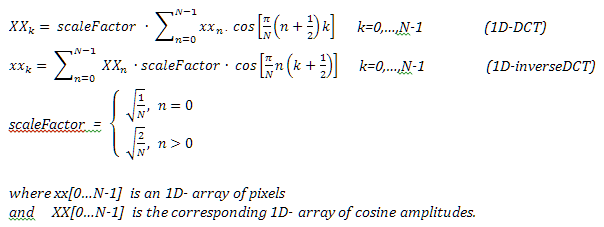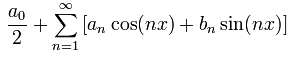• ## Basics of the Discrete Cosine Transform

The Discrete Cosine Transform DCT is derived from the Discrete Fourier Transform DFT.
You can find an intuitive introduction into Charles Fourier's harmonic analysis here: Lecture on the Fourier Transform
The summary of this lecture is:
DCT splits any periodic oscillation into a sum of harmonic oscillations of multiples of a fundamental frequency.
The inverse DCT is the reversal of this decomposition, which means the reconstruction of an arbitrary waveform through addition of harmonic oscillations.

Problem: At first sight there are no periodic oscillations inside an image. There are just rows and columns of pixels, which do not repeat at all.
Solution: We virtually repeat any row infinite times, so to say to virtually continue each row after its rightmost pixel with the row's leftmost pixel and so on. So we imagine having replaced our pixel row by an infinite periodic oscillation that the DCT can split into a sum of harmonic oscillations.

In terms of image processing, the DCT maps an array of equidistant gray values `xx[0...N-1]` into the frequency domain, that is to say into an array of the same length of amplitudes `XX[0...N-1]` of `N` cosine basis functions.
The inverse DCT (synonym: DCT back transform) can exactly restore `xx` from `XX` but in most cases varied filtering is applied by restricting the back transform to a limited set of frequencies.

Important: In the following formulas and in all following code snippets
the lowercase `xxk` and `xx[k]` contain input or output gray values whereas
the uppercase `XXn` and `XX[n]` contain cosine amplitudes.Further readings: Wikipedia: DCT and Wikipedia: DFT and Miszalok: Lecture on the Fourier Transform

```//C#-Code of the Discrete Cosine Transform DCT and of its back transform:
//xx[0...N-1] = spatial domain input/output array, XX[0...N-1] = frequency domain array
private void DCT( float[] xx, float[] XX )
{ int k, n, N = xx.Length;
float sum;
float scaleFactor0 = (float)Math.Sqrt( 1.0 / N );
float scaleFactor  = (float)Math.Sqrt( 2.0 / N );
double piN =   Math.PI / N;
for ( k=0; k < N; k++ )
{ sum = 0;
for ( n=0; n < N; n++ ) sum += xx[n] * (float)Math.Cos( piN*(n+0.5)*k );
if ( k==0 ) XX[k] = sum * scaleFactor0;
else        XX[k] = sum * scaleFactor ;
}
}
private void DCTBack( float[] XX, float[] xx )
{ int k, n;
float sum;
float scaleFactor0 = (float)Math.Sqrt( 1.0 / N );
float scaleFactor  = (float)Math.Sqrt( 2.0 / N );
double piN = Math.PI / N;
for ( k = 0; k < N; k++ )
{ sum = XX * scaleFactor0;
for ( n = 1; n < N; n++ ) sum += XX[n] * scaleFactor * (float)Math.Cos( piN*n*(k+0.5) );
xx[k] = sum;
}
}```
• ## 1D-DCT and its Back Transform

Experiments:

1. Observe how the change of one input value changes all amplitudes !
Take notice of the permanent identity of the input values and the back-transformed values !

2. Drag the sliders arbitrarily.

3. Drag all sliders to 0.

4. Drag all sliders to 100.

5. Drag 1., 3., 5., etc. sliders to 0 and 2., 4., 6., etc. sliders to 100. Result: frequencies 2, 4, 6, 8, 10 are almost 0.

6. Drag 1+2, 5+6, 9+10 to 100 and all others to 0. Result: frequencies 2, 4, 6, 8, 10 are almost 0.

7. It is easy to install, run and zoom this sample program out of the browser:
Right click the sample → `Install DCT1D Application onto this computer...` → Uncheck `Start menu`, check `Desktop``OK` → Click the `DCT1D Application` icon.
To uninstall `DCT1D` from your computer, just right click the running application → `Remove this application...``Yes`.

```//The complete Discrete Cosine Transform back transform.
//xx[0...N-1] = spatial domain input/output array, XX[0...N-1] = frequency domain array
private void DCTBack( float[] XX, float[] xx )
{ int k, n;
float sum;
float scaleFactor0 = (float)Math.Sqrt( 1.0 / N );
float scaleFactor  = (float)Math.Sqrt( 2.0 / N );
double piN = Math.PI / N;
for ( k = 0; k < N; k++ )
{ sum = XX * scaleFactor0;
for ( n = 1; n < N; n++ )
sum += XX[n] * scaleFactor * (float)Math.Cos( piN*n*(k+0.5) );
xx[k] = sum;
}
}```
• ## 1D-DCT with a Limited Back Transform

Experiments:

1. Observe the differences of the original and the back-transformed values whenever you deselect frequencies !

2. Observe the red line which visualizes the result of the back-transformation.

3. Make the same experiments as with the previous applet above.

```//Just one line changed within the DCT back transform.
//xx[0...N-1] = spatial domain input/output array, XX[0...N-1] = frequency domain array
private void DCTBack( float[] XX, float[] xx )
{ int k, n;
float sum;
float scaleFactor0 = (float)Math.Sqrt( 1.0 / N );
float scaleFactor  = (float)Math.Sqrt( 2.0 / N );
double piN = Math.PI / N;
for ( k = 0; k < N; k++ )
{ sum = XX * scaleFactor0;
for ( n = 1; n < N; n++ )
if ( cb[n].IsChecked == true ) sum += XX[n] * scaleFactor * (float)Math.Cos( piN*n*(k+0.5) );
xx[k] = sum;
}
}```
• ## 2D-DCT of Monochrome Images

Color image processing is slow. Any pixel has to be treated 3 times because of its different red, green and blue values.
But pixels of a monochrome image have identical red, green and blue bytes.
Monochrome image processing is therefore much easier and faster.
We transform and back transform just the red values (forget about the identical green and blue ones) and in the end we copy the back transformed values threefold into the red, green and blue bytes of the output pixels.

Experiments:

1. In order to obtain small slider increments and decrements click on the horizontal guide rail right or left and finally click the valve itself.

2. Lower HorizHighestFrequ down to 3.

3. Lower VerticHighestFrequ down to 3.

4. Increase HorizLowestFrequ to 4, 5, 6 .....

5. Increase VerticLowestFrequ to 4, 5, 6 ....

6. Shift all sliders to their minimum and increment both HighestFrequ-Sliders stepwise to 4, 5, 6 etc.

7. a) Shift VerticLowestFrequ to 1 and VerticHighestFrequ to maximum.
b) Shift both horizontal frequencies to minimum.
c) Increment HorizLowestFrequ to 2, 3, 4, etc.
d) HorizHighestFrequ automatically increments to 5, 6, 7 etc.
e) The narrow horizontal bandwidth results in strange artifacts.

8. a) Shift HorizLowestFrequ to 1 and HorizHighestFrequ to maximum.
b) Try the same as 6. b), c), d) e) in vertical direction.

9. Open an arbitrary small image. (Color images will be truncated to red and converted to monochromes.)

10. Make experiment 7 of the applet "1D-DCT and its Back Transform" above.

• ## 2D-DCT of Color Images

******************************************************************************************************
Q: What is the Fourier series ?

 A: A function F(x) is represented as the sum of sine- and cosine-waves: F(x) =******************************************************************************************************
Q: What is the cosine series ? Comparison to Fourier series ?
A: Fourier: Base functions: cosine and sine.
Cosine: Base functions: just cosine.
Advantage of cosine: No complex additions and multiplications necessary → less complicated algorithm
Both need nearly the same considerable computational time.
******************************************************************************************************
Q: What sorts of image filters are possible using the inverse DFT or the inverse DCT ?
A;

1. Lowpass filtering by leaving out high frequencies

2. Highpass filtering by leaving out low frequencies

3. Bandpass filtering by leaving out low and high frequencies

1. Easy filtering of images that are derived from frequency measurements ( CT, NMR, Radar, Sonar etc ).

2. Easy removing of known interference frequencies.

3. Most graphic boards have fast integrated circuits specialized on DCT.

4. Well suited for lossy image compression

1. Extensive computation time without specialized hardware.

2. Leaving out important frequencies can produce severe artifacts.

******************************************************************************************************
Q: Computing time of DFT and DCT of an NxN image ? What is FFT ?
Both DFT and DCT and their inverse transforms run 3 nested for-loops 0...N which results in N3 operations.
The Fast Fourier Transform FFT reduces N3 to log2N*N2 operations.
Drawback: The FFT assumes N being a power of 2 such as 128, 256, 512 etc.
******************************************************************************************************
Q: JPG is a lossy image compression standard basing on DCT. Why is it so fast ?
A: The image is subdivided into small 8x8 pixel blocks which undergo DCT.
The resulting data for all 8x8 blocks is further compressed by Huffmann encoding.
Nowadays all modern graphic chips contain a processor pipeline specialized in DCT and Huffmann encoding.